Next: Active Filters Up: Mathematical Operations Previous: Differentiation Circuit

### Integration Circuit

Integration is obtained by reversing the resistor and the capacitor as shown in figure 6.11. The capacitor is now in the feedback loop.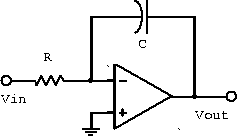Figure 6.11:  Integration circuit.

Analysis gives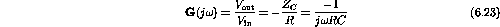or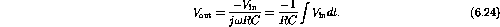Using dV/dt=I/C gives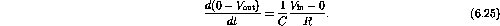and thus the same result as above.

The frequency response is shown in figure 6.12.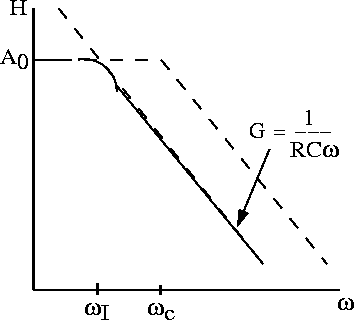Figure 6.12:  Integration circuit frequency response.

We can combine the above inverting, summing, offset, differentiation and integration circuits to build an analog computer that can solve differential equations. However, today, the differentiators and integrators are mainly used to condition signals.

Doug Gingrich
Tue Jul 13 16:55:15 EDT 1999